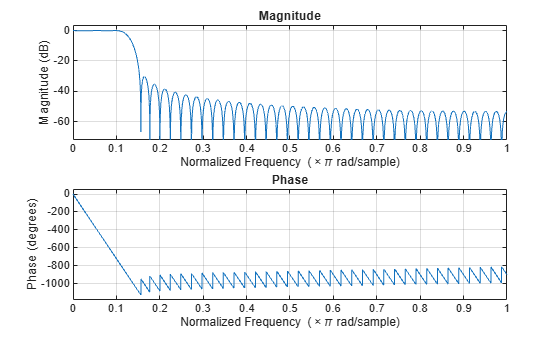# fvtool

Plot frequency response of filter

## Syntax

``fvtool(rcfilter)``
``fvtool(rcfilter,options)``
``fvtool(____,Name,Value)``

## Description

example

````fvtool(rcfilter)` plots the magnitude response of the specified filter.```
````fvtool(rcfilter,options)` plots the response that is specified by `options`.For example, to plot the impulse response of the specified filter, set `options` to `'impulse'`.fvtool(rcfilter,'impulse');```
````fvtool(____,Name,Value)` specifies additional options using one or more name-value arguments in addition to any input argument combination from the previous syntaxes.For more input options, see the FVTool function.```

## Examples

collapse all

Create a square-root-raised-cosine (SRRC) transmit filter System object™, and then plot the filter response. The results show that the linear filter gain is greater than unity. Specifically, the passband gain is greater than 0 dB.

```txfilter = comm.RaisedCosineTransmitFilter; fvtool(txfilter)```Obtain the filter coefficients by using the `coeffs` object function and adjust the filter gain to unit energy.

`b = coeffs(txfilter);`

Because a filter with unity passband gain must have filter coefficients that sum to 1, set the linear filter gain to the inverse of the sum of the filter tap coefficients, `b.Numerator`.

`txfilter.Gain = 1/sum(b.Numerator);`

Verify that the resulting filter coefficients sum to 1.

```bNorm = coeffs(txfilter); sum(bNorm.Numerator)```
```ans = 1.0000 ```

Plot the filter frequency response again. The results now show that the passband gain is 0 dB, which is unity gain.

`fvtool(txfilter)`## Input Arguments

collapse all

Filter, specified as one of these System objects.

Filter analysis options, specified as one of these values:

• `'magnitude'` –– Magnitude response

• `'phase'` –– Phase response

• `'freq'` –– Frequency response

• `'grpdelay'` –– Group delay

• `'phasedelay'` –– Phase delay

• `'impulse'` –– Impulse response

• `'step'` –– Step response

• `'polezero'` –– Pole zero plot

• `'coefficients'` –– Coefficients vector

• `'info'` –– Filter information

• `'magestimate'` –– Magnitude response estimate

• `'noisepower'` –– Round-off noise power spectrum

### Name-Value Arguments

Specify optional pairs of arguments as `Name1=Value1,...,NameN=ValueN`, where `Name` is the argument name and `Value` is the corresponding value. Name-value arguments must appear after other arguments, but the order of the pairs does not matter.

Before R2021a, use commas to separate each name and value, and enclose `Name` in quotes.

Example: `fvtool(rcfilter,'Arithmetic','single')`

Sampling rate, specified as a scalar. This value, Fs, determines the Nyquist interval [–Fs/2, Fs/2] in which the function shows the frequency response of the filters in the channelizer.

Data Types: `single` | `double`

Specify the arithmetic used during analysis. The analysis tool assumes a double-precision filter when the arithmetic input is not specified and the filter System object is unlocked. The `'Arithmetic'` property set to `'Fixed'` applies only to filter System objects with fixed-point properties.

When you specify this argument as `'Fixed'`, the function plots the double-precision reference filter and the quantized version of the filter. The function uses the `CoefficientsDataType` property of the respective filter System object to create the quantized version of the filter analysis values in `options` except for these:

• `'magestimate'` –– Magnitude response estimate

• `'noisepower'` –– Round-off noise power spectrum

For these two analysis options, the function uses all of the fixed-point settings to analyze the quantized version of the filter.

Data Types: `char` | `string`

## Version History

Introduced in R2013b